Quantitative MCQ - 29

# Quantitative MCQ - 29

Test Description

## 30 Questions MCQ Test Quantitative Aptitude for Competitive Examinations | Quantitative MCQ - 29

Quantitative MCQ - 29 for Quant 2022 is part of Quantitative Aptitude for Competitive Examinations preparation. The Quantitative MCQ - 29 questions and answers have been prepared according to the Quant exam syllabus.The Quantitative MCQ - 29 MCQs are made for Quant 2022 Exam. Find important definitions, questions, notes, meanings, examples, exercises, MCQs and online tests for Quantitative MCQ - 29 below.
Solutions of Quantitative MCQ - 29 questions in English are available as part of our Quantitative Aptitude for Competitive Examinations for Quant & Quantitative MCQ - 29 solutions in Hindi for Quantitative Aptitude for Competitive Examinations course. Download more important topics, notes, lectures and mock test series for Quant Exam by signing up for free. Attempt Quantitative MCQ - 29 | 30 questions in 30 minutes | Mock test for Quant preparation | Free important questions MCQ to study Quantitative Aptitude for Competitive Examinations for Quant Exam | Download free PDF with solutions
 1 Crore+ students have signed up on EduRev. Have you?
Quantitative MCQ - 29 - Question 1

### Directions (1-5): What should come in place of question mark (?) in the following number series?  567, 295, 159, 91, 57, ?

Detailed Solution for Quantitative MCQ - 29 - Question 1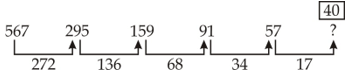Quantitative MCQ - 29 - Question 2

### Directions (1-5): What should come in place of question mark (?) in the following number series? 76, 115, 64, 103, 52, ?

Detailed Solution for Quantitative MCQ - 29 - Question 2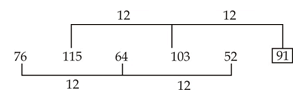Quantitative MCQ - 29 - Question 3

### Directions (1-5): What should come in place of question mark (?) in the following number series? 963, 927, 855, 747, 603, 423, ?

Detailed Solution for Quantitative MCQ - 29 - Question 3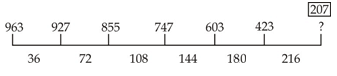Quantitative MCQ - 29 - Question 4

Directions (1-5): What should come in place of question mark (?) in the following number series?

23, 15, 22, 58, 224, ?

Detailed Solution for Quantitative MCQ - 29 - Question 4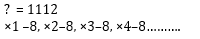Quantitative MCQ - 29 - Question 5

Directions (1-5): What should come in place of question mark (?) in the following number series?

7, 42, 504, ?, 217728, 6531840

Detailed Solution for Quantitative MCQ - 29 - Question 5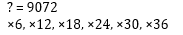Quantitative MCQ - 29 - Question 6

Directions (6-10): In the following questions two equations numbered (I) and (II) are given. You have to solve both equations and give answer

I. x² + 15x + 56 = 0

II. y² + 17y + 72 = 0

Detailed Solution for Quantitative MCQ - 29 - Question 6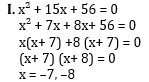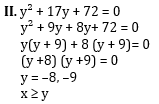Quantitative MCQ - 29 - Question 7

Directions (6-10): In the following questions two equations numbered (I) and (II) are given. You have to solve both equations and give answer

I. 2x² – 9x + 10 = 0

II. 9y² - 57y + 88 = 0

Detailed Solution for Quantitative MCQ - 29 - Question 7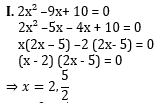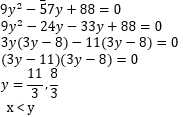Quantitative MCQ - 29 - Question 8

Directions (6-10): In the following questions two equations numbered (I) and (II) are given. You have to solve both equations and give answer

I. 3x + 2y = 7√14

II. 10x + 14y =38√14

Detailed Solution for Quantitative MCQ - 29 - Question 8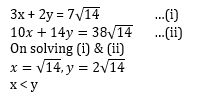Quantitative MCQ - 29 - Question 9

Directions (6-10): In the following questions two equations numbered (I) and (II) are given. You have to solve both equations and give answer

I. x² - 11x + 28 = 0

II. y² + 3y – 40 = 0

Detailed Solution for Quantitative MCQ - 29 - Question 9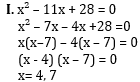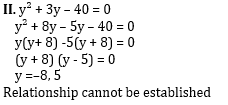Quantitative MCQ - 29 - Question 10

Directions (6-10): In the following questions two equations numbered (I) and (II) are given. You have to solve both equations and give answer

I. x² = 361

II. y = √441

Detailed Solution for Quantitative MCQ - 29 - Question 10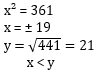Quantitative MCQ - 29 - Question 11

Directions (Q.11 - 15) : What will come in place of question mark (?) in the following number series?

26, 12, 10, 16, ?

Detailed Solution for Quantitative MCQ - 29 - Question 11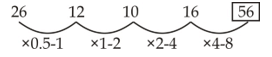Quantitative MCQ - 29 - Question 12

Directions (Q.11 - 15) : What will come in place of question mark (?) in the following number series?

82, 107, 207, 432, 832, ?

Detailed Solution for Quantitative MCQ - 29 - Question 12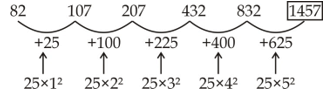Quantitative MCQ - 29 - Question 13

Directions (Q.11 - 15) : What will come in place of question mark (?) in the following number series?

122, 213, 340, 509, ?, 997

Detailed Solution for Quantitative MCQ - 29 - Question 13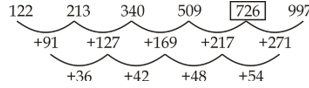Quantitative MCQ - 29 - Question 14

Directions (Q.11 - 15) : What will come in place of question mark (?) in the following number series?

25, 37.5, 56.25, ?, 126.5625

Detailed Solution for Quantitative MCQ - 29 - Question 14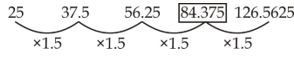Quantitative MCQ - 29 - Question 15

Directions (Q.11 - 15) : What will come in place of question mark (?) in the following number series?

10, 50, 94, 142, 194, ?

Detailed Solution for Quantitative MCQ - 29 - Question 15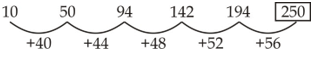Quantitative MCQ - 29 - Question 16

In a mixture of milk and water the proportion of water by weight was 75%. If in the 60 gms mixture 15 gms water was added, what would be the percentage of water in the new mixture?

Detailed Solution for Quantitative MCQ - 29 - Question 16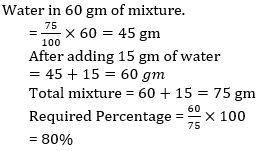Quantitative MCQ - 29 - Question 17

If 36 persons are engaged on a piece of work, the work can be completed in 40 days. After 32 days, only 3/4th of the work was completed. How many more persons are required to complete the work on time?

Detailed Solution for Quantitative MCQ - 29 - Question 17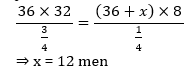Quantitative MCQ - 29 - Question 18

The simple interest accrued on an amount of Rs. 22,500 at the end of four years is Rs. 10,800. What would be the compound interest accrued on the same amount at the same rate at the end of two years?

Detailed Solution for Quantitative MCQ - 29 - Question 18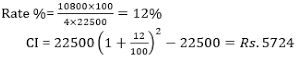Quantitative MCQ - 29 - Question 19

If the length of a rectangular field is increased by 20% and the breadth is reduced by 20%, the area of the rectangle will be 192m2. What is the area of the original rectangle?

Detailed Solution for Quantitative MCQ - 29 - Question 19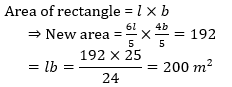Quantitative MCQ - 29 - Question 20

Directions (20-24): What should come in place of question mark (?) in the following number series?

1, 1, 3, 9, 13, 65, 71, ?, 505

Detailed Solution for Quantitative MCQ - 29 - Question 20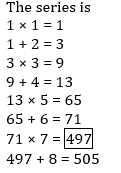Quantitative MCQ - 29 - Question 21

Directions (20-24): What should come in place of question mark (?) in the following number series?

1, 2, 6, 42, ?, 3263442

Detailed Solution for Quantitative MCQ - 29 - Question 21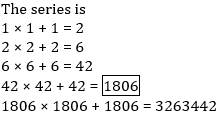Quantitative MCQ - 29 - Question 22

Directions (20-24): What should come in place of question mark (?) in the following number series?

56, 57, 48, 73, 24, 105, ?

Detailed Solution for Quantitative MCQ - 29 - Question 22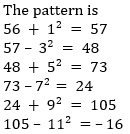Quantitative MCQ - 29 - Question 23

Directions (20-24): What should come in place of question mark (?) in the following number series?

3, 1, 3, 0.75, 3, 0.6, 3, ?

Detailed Solution for Quantitative MCQ - 29 - Question 23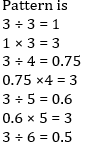Quantitative MCQ - 29 - Question 24

Directions (20-24): What should come in place of question mark (?) in the following number series?

2, 5, 10, 17, 28, 41, 58, ?

Detailed Solution for Quantitative MCQ - 29 - Question 24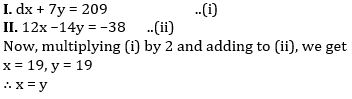Quantitative MCQ - 29 - Question 25

Directions (25-29): In the following questions two equations numbered I and II are given. Solve the equation and answer the question if:

I. 4x + 7y = 209
II. 12x – 14y = -38

Detailed Solution for Quantitative MCQ - 29 - Question 25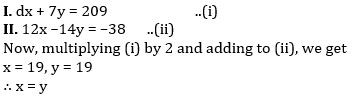Quantitative MCQ - 29 - Question 26

Directions (25-29): In the following questions two equations numbered I and II are given. Solve the equation and answer the question if:

I. (17)2 + 144 ÷ 18 = x
II. (26)2 – 18 × 21 = y

Detailed Solution for Quantitative MCQ - 29 - Question 26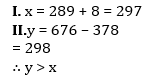Quantitative MCQ - 29 - Question 27

Directions (25-29): In the following questions two equations numbered I and II are given. Solve the equation and answer the question if:

I. x2– 11x + 24 = 0
II. 2y2 – 9y + 9 = 0

Detailed Solution for Quantitative MCQ - 29 - Question 27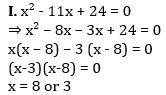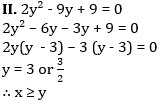Quantitative MCQ - 29 - Question 28

Directions (25-29): In the following questions two equations numbered I and II are given. Solve the equation and answer the question if:

I. 18x2 + 18x + 4 = 0
II. 12y2 + 29y + 14 = 0

Detailed Solution for Quantitative MCQ - 29 - Question 28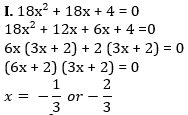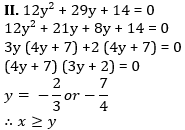Quantitative MCQ - 29 - Question 29

Directions (25-29): In the following questions two equations numbered I and II are given. Solve the equation and answer the question if:

I. 8x2 + 6x = 5
II. 12y2 – 22y + 8 = 0

Detailed Solution for Quantitative MCQ - 29 - Question 29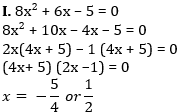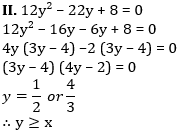Quantitative MCQ - 29 - Question 30

What is the ratio of the total number of votes polled in Chandani Chowk and Purani Delhi together in 2008 to the total number of votes polled in these two constituencies together in 2013 ?

Detailed Solution for Quantitative MCQ - 29 - Question 30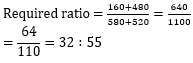## Quantitative Aptitude for Competitive Examinations

33 videos|34 docs|140 tests
 Use Code STAYHOME200 and get INR 200 additional OFF Use Coupon Code
Information about Quantitative MCQ - 29 Page
In this test you can find the Exam questions for Quantitative MCQ - 29 solved & explained in the simplest way possible. Besides giving Questions and answers for Quantitative MCQ - 29, EduRev gives you an ample number of Online tests for practice

## Quantitative Aptitude for Competitive Examinations

33 videos|34 docs|140 tests